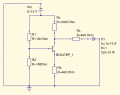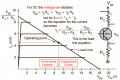# Back to basics - BJT transistor BC547B (Solved)

#### PsySc0rpi0n

Joined Mar 4, 2014
1,706
Hello.

I'm trying to get back to the basics of BJT transistors. This is no homework. Just me trying to get back to the basics.
I want to write the net equations and node equations but there are a few things that are forgotten and I'm struggling a bit to get them back to my mind.
I'm using Qucs to simulate the circuit. I'm not sure it's files are compatible with LTSpice which I know is probably more common around here than Qucs but I'll upload the files here.

I want to calculate all resistor's voltage drops and currents of interest so that I can compare them with Qucs results.
This is the circuit.
I think I can write the following equations but I'm not sure they are correct

$$\displaystyle{I_{R_{1}} = I_{R_{2}} + I_{b} }$$
$$\displaystyle{12V - V_{R_{1}} - V_{R_{2}} = 0}$$
$$\displaystyle{0.7V = V_{R_{2}} - V_{R_{3}}}$$
$$\displaystyle{12V = V_{R_{c}} + V_{R_{L}} + V_{LED}}$$
$$\displaystyle{12V = V_{R_{c}} + V_{CE} + V_{R_{E}}}$$

Now, are these equations all good? Can I discard anyone or I'm going to need them all?#### Attachments

• 1.8 KB Views: 8

#### Marley

Joined Apr 4, 2016
501
All good except the base-emitter voltage will be nearer 0.65V.
So: 0.65V=VR2−VR3

•PsySc0rpi0n

#### ericgibbs

Joined Jan 29, 2010
18,007
hi Psy.
Is this LTS sim any help.?

E

#### Attachments

•PsySc0rpi0n

#### PsySc0rpi0n

Joined Mar 4, 2014
1,706
@Marley thanks for letting me know.

@ericgibbs yeah, I have pretty much the same results at Qucs, so, those confirm. The struggle is with the equations, but apparently they are ok.

@Jony130 yeah, I think that's what I was doing right there but I started from the most basic equations.

#### Audioguru again

Joined Oct 21, 2019
6,155
The 1M values of the base bias resistors R3 and R4 are much too high. Use 20k and 10k.

#### LvW

Joined Jun 13, 2013
1,669
Just a rough calculation (ignoring, as a first step, the base current):
* The base voltage will be app. Vb=6V (voltage division, 2 equal R),
* So the emitter voltage should be (when the BJT works as desired) Ve=app. 5.3V
* Now you can calculate the current Ie, which is app. identical to the current Ic (at the collector node).
* Calculating the corresponding collector potential Vc you will see that the BJT cannot work as desired.

#### PsySc0rpi0n

Joined Mar 4, 2014
1,706
So, using the numbers of my circuit, I'm not getting anywhere near what the simulation returns.

I even tried to solve this by hand on paper but with 4 equations it gets tricky. Seems that I'm going round and round. So I plugged the equations into the calculator and got different results from Qucs and also if I plug the same values to @Jony130 example equation for $$\displaystyle{I_{b}}$$

$$\displaystyle{\begin {vmatrix} V_{cc} = I_{1}\cdot R_{1} + I_{2} \cdot R_{2}\\ I_{1} \cdot R_{1} = I_{b} + I_{2} \cdot R_{2}\\ I_{2} \cdot R_{2} = V_{bE} + I_{E} \cdot R_{E}\\ I_{b} = \frac{I_{E} \cdot R_{E}}{\beta + 1} \end{vmatrix} }$$

Calculator did the hardwork but things doesn't match with Qucs or LTSpice from @ericgibbs.

I got
I1 = 6.0133μA
I2 = 5.9867μA
IE = 11.6016mA
Ib = 0.02655mA

So, for R1 = 1MΩ, an with I1 = 6μA, I would have 6V drop across R1. But Qucs says 1.78868V.
If I pulg the valaues into @Jony130 equation example, I get:
$$\displaystyle{I_{b} = \frac{1M\Omega\cdot 12V - 0.65V\left (1M\Omega+1M\Omega\right )}{\left (200 + 1\right )\cdot 460 \cdot \left ( 1M\Omega + 1M\Omega\right ) + \left ( 1M\Omega \cdot 1M\Omega \right )} = 9.0301 \mu A}$$

Last edited:

#### PsySc0rpi0n

Joined Mar 4, 2014
1,706
The 1M values of the base bias resistors R3 and R4 are much too high. Use 20k and 10k.
The current on the base will be amplified beta times, right? So, I want tiny currents there.
Anyway, the point is to calculate whatever values I get there an check them with Qucs/LTSpice

#### Jony130

Joined Feb 17, 2009
5,457

I mean this one:

I1*R1 = Ib + I2*R2

Do you see it?

Also notice that I1 = Ib+I2

I'm not getting anywhere near what the simulation returns
How close do you want to match the simulation results?

Because 9μA for a base current looks good enough for me.

Ie ≈ 1.8mA
Vb ≈ 0.65V + 1.8mA*0.46kΩ ≈ 1.47V
I2 = 1.48μA
I1 = 9μA + 1.48μA ≈ 10.5μA

Last edited:

#### Audioguru again

Joined Oct 21, 2019
6,155
Did you buy hundreds of BC547B transistors, measure the beta of them all then maybe find one that has your selected base current of 9uA? Its datasheet says its beta could be 220 or could be 500. Don't you want every BC547B transistor to work?
Then the current in the base voltage divider resistors must be about 10 times the base current so that the base voltage does not change much when the beta is different.

I biased the base at 2.54V so that the collector can have the widest unloaded voltage swing, then the collector is 7.9V at idle.

#### click_here

Joined Sep 22, 2020
548
I did the same thing a few years back - I bought the book "The Art of Electronics", and it's lab book, worked my way through it, and rediscovered a love of analogue electronics.

•PsySc0rpi0n

#### sparky 1

Joined Nov 3, 2018
735
page 2 small signal charecteristics
The noise figure listed is 2dB for Ic of 200uA or 0.2mA at 5V
the 2mA with 1 kHz looks interesting to explore just what is the hFE current gain they tend to vary.

https://www.onsemi.com/pdf/datasheet/bc546-d.pdf

Last edited:

#### MrChips

Joined Oct 2, 2009
29,217
Firstly, remove RL and D1 and attempt to solve it without a load attached.

Imagine an operating load line drawn on a I-V graph.Extreme operating points are (12V, 0A) on the right (VCE axis) and (0V, (12/1.46k)A) on the left (Ic axis).
In other words the max collector current is (12V/1.46k)A = 8mA
Voltage across Re = 8mA x 460Ω = 3.7V
With Vb = 6V, the transistor is in saturation mode.

#### PsySc0rpi0n

Joined Mar 4, 2014
1,706

I mean this one:

I1*R1 = Ib + I2*R2

Do you see it?

Also notice that I1 = Ib+I2

How close do you want to match the simulation results?

Because 9μA for a base current looks good enough for me.

Ie ≈ 1.8mA
Vb ≈ 0.65V + 1.8mA*0.46kΩ ≈ 1.47V
I2 = 1.48μA
I1 = 9μA + 1.48μA ≈ 10.5μA
I see it. I meant it to be:

$$\displaystyle{I_{1} = I_{b} + I_{2}}$$

Let me see where does this takes me. I'll try to do this by hand because I don't have a calculatir here right now.

About the current, well, as soon as possible. The point I meant to mention was that I was getting pretty wrong values.

#### PsySc0rpi0n

Joined Mar 4, 2014
1,706
Did you buy hundreds of BC547B transistors, measure the beta of them all then maybe find one that has your selected base current of 9uA?
I don't understand the irony about buying hundreds of transistors and do what you said plus finding one to have 9μA.

Its datasheet says its beta could be 220 or could be 500.
Datasheet I saw, said a gain netween 20 and 200, iirc. But I can try to find the same pdf to confirm. So, pretty much different from what you have. I assumed the gain at 200.

Don't you want every BC547B transistor to work?
And of course I want every transistor to work. Why sohuldn't they work? Aren't they meant to work? I don't understsand, sir. I'm completely lost.

Then the current in the base voltage divider resistors must be about 10 times the base current so that the base voltage does not change much when the beta is different.

I biased the base at 2.54V so that the collector can have the widest unloaded voltage swing, then the collector is 7.9V at idle.
Ok, I'll have that into account. But for now, I just want to get the equations right.

1 step at a time.#### ericgibbs

Joined Jan 29, 2010
18,007
hi Psy.
It is a typical case of posters not reading your original post correctly.

All you were asking for, is confirmation of your calculations, compared to the simulation results, not how to design a BJT amplifier or read a datasheet.

E

•PsySc0rpi0n

#### PsySc0rpi0n

Joined Mar 4, 2014
1,706
I did the same thing a few years back - I bought the book "The Art of Electronics", and it's lab book, worked my way through it, and rediscovered a love of analogue electronics.
I have the same book. I bought back then and I read it someitmes. But in this case, I really need to get back to the basics of finding equations and etc.#### PsySc0rpi0n

Joined Mar 4, 2014
1,706
hi Psy.
It is a typical case of posters not reading your original post correctly.

All you were asking for, is confirmation of your calculations, compared to the simulation results, not how to design a BJT amplifier or read a datasheet.

E
Yes, most probably that's the case.

Anyway, I tried to do the math on paper and it gets pretty nasty in terms of long equations, and the probability for errors, rises like exponentially.

I took my 4 equations, with the second one fixed as @Jony130 pointed out. My mistake with that second equations was that my brain was focused in voltages, hence the R multiplied by the currents on that second equation. If I remove the Rs from that equation, it's the same as @Jony130 pointed.

By hand, I started by replacing the 4th equation in the 2nd equation.
Then, I replaced the 3rd equation, also in the 2nd equation, and I solved for $$\displaystyle{I_{E}}$$.
I'm not going to type here all the steps in Latex because that would take too much time but I got 1.406mA for $$\displaystyle{I_{E}}$$. @Jony130 got 1.8mA. Not sure my result is correct or if 0.4mA is a considerable difference.

Anyway, I'll uploda 2 pictures of all the steps I took.
Warning: This might not be suitable for people with heart rate conditions (I'm joking, of course).

#### LvW

Joined Jun 13, 2013
1,669
I got 1.406mA for $$\displaystyle{I_{E}}$$. @Jony130 got 1.8mA. Not sure my result is correct or if 0.4mA is a considerable difference.
Perhaps you are interested in the reason for this difference.
The reason is in your design which is a bad one. Let me explain:
Normally, the resistive voltage divider at the base is selected to be as low-resistive as possible because each good design should provide the required base voltage - independend on the pretty large tolerances of the current gain B (resp. beta).
(Rule of thumb: The base current should be max. 10% of the current through the divider chain).
The lower limit for both resistors is set by the allowed power consumption as well as the input resistance of the whole stage.

In your design, we see two resistors - each of 1 MegOhm.
As a result, the voltage at the base is strongly dependent on the base current which is different for the various transistors.
Example: I have simulated your circuit with the given values and a BC108 macro model.
The emitter current was 1.59mA.
For a better design with a low-resistive voltage divider and a suitable emitter resistor you will have an emitter current which will be much less dependent on the selected transistor type.